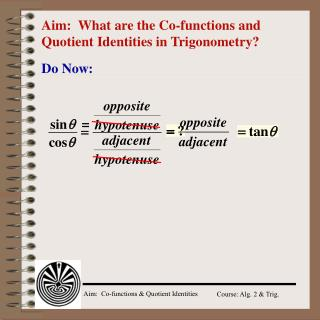Download PresentationAim: What are the Co-functions and Quotient Identities in Trigonometry?

Aim: What are the Co-functions and Quotient Identities in Trigonometry? - PowerPoint PPT Presentation

Aim: What are the Co-functions and Quotient Identities in Trigonometry?. Do Now:. =. Reciprocal Identities. Quotient Identities. Use in verification of identities and in numerical calculations. Verify the Identity. A trig identity is an equation that is true for all values of variable.I am the owner, or an agent authorized to act on behalf of the owner, of the copyrighted work described.
Download PresentationAim: What are the Co-functions and Quotient Identities in Trigonometry?

Download Policy: Content on the Website is provided to you AS IS for your information and personal use and may not be sold / licensed / shared on other websites without getting consent from its author.While downloading, if for some reason you are not able to download a presentation, the publisher may have deleted the file from their server.

- - - - - - - - - - - - - - - - - - - - - - - - - - E N D - - - - - - - - - - - - - - - - - - - - - - - - - -
Presentation Transcript
1. Aim: What are the Co-functions and Quotient Identities in Trigonometry? Do Now: =

2. Reciprocal Identities Quotient Identities Use in verification of identities and in numerical calculations

3. Verify the Identity A trig identity is an equation that is true for all values of variable. verify

4. Reciprocal Identity Quotient Identity What does simplifying mean? Simplifying a trig expressions usually means expressing in terms of sine and cosine.

5. usually written (sin )2 = sin2 Model Problems Write an expression in terms of sin , cos , or both. Simplify whenever possible. 1. sec  • cot  2.

6. Calculator: 1 ÷ tan ) x2 ENTER 30 Display: 3 Model Problems Find the exact numerical value for each: 1. cot2 30º

7. Model Problems Find the exact numerical value for each: 2. cot 45º • csc 45º tan 45º = 1 cot 45º= 1 cot 45º • csc 45º 1 •

8. Co- sin  = cos(90º – ) cos  = sin(90º – ) sine  cosine Co- tan  = cot(90º – ) cot  = tan(90º – ) tangent  cotangent Co- sec  = csc(90º – ) csc  = sec(90º – ) secant  cosecant Trigonometry Co-functions An trigonometric function of an acute angle is equal to the co-function of its complement.

9. Co- sin  = cos(90º – ) cos  = sin(90º – ) Co- tan x = cot(90º – x) cot x = tan(90º – x) Model Problems Write in terms of the co-function the sine of 30o. • If x and y are the measures of two acute angles and tan x = cot y, then • x = y + 90 2) x = y – 90 • 3) x = 90 – y 4) y =x – 90 y = (90º – x)

10. Model Problem If sin x = cos(x + 20º) and x and (x + 20º) are the measure of two acute angles, find x. sine and cosine are co-functions If the sine of an acute angle is equal to the cosine of another acute angle, the angles must be complementary. x + 20º + x= 90º 2x + 20º = 90º 2x = 70º x = 35º x + 20º = 55º √ cos 55º = .57357… sin 35º = .57357…

11. Which expression is NOT equal to the other three expressions? Model Problems